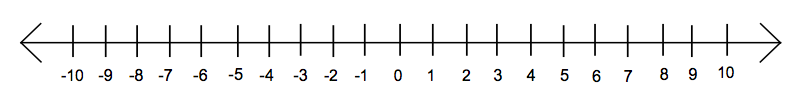## 1.3 Math Review for Preparatory Chemistry

• Place Value in the Decimal System
• Positive and Negative Numbers: Addition and Subtraction
• Positive and Negative Numbers: Multiplication and Division
• Algebra

## Place Value in the Decimal System

Recall that in the decimal system, each place in the number has a name and a multiplier value.  For instance, to the left of the decimal point are the ones place, the tens place, the hundreds place, and so on.  To the right of the decimal point are the tenths place, the hundredths place, the thousandths place, etc.  The value of a digit (0, 1, 2, 3, 4, 5, 6, 7, 8, 9) depends on its place in the number.  For instance, a 3 in the ones place is worth three, but in the tens place it is worth thirty and in the hundredths place is worth three-hundredths.

### Determining place value in the Decimal system

Identify the place value for each of the digits in the number 401.725.

a. 4

b. 0

c. 1

d. 7

e. 2

f. 5

## Positive and Negative Numbers: Addition and Subtraction

One way to think about positive and negative numbers is to use a number line like the one shown below.  This may help you recall and understand the rules for adding, subtracting, multiplying, and dividing numbers to get answers with the correct absolute values and signs.Addition of a positive number means moving to the right on the number line, and subtraction of a positive number is moving to the left.   When the number added or subtracted has a negative sign, it means moving in the opposite direction.  Use the number line to confirm the rules for adding and subtracting numbers:

1. For addition, the order of the numbers doesn’t matter. For subtraction, the order does matter.
2. The sum of two positive numbers is the sum of their absolute values, and the sign of the answer is positive.
3. The sum of two negative numbers is the sum of their absolute values, and the sign of the answer is negative.
4. The sum of a positive number and a negative number is the difference between their absolute values, and the sign of the answer is the same as the sign of the number with the larger absolute value.
5. Subtracting a negative number is equivalent to adding.

### Addition and Subtraction of Numbers

Give the answers to the following:

a. -13 + 23 = ____

b. -8 – (-4) = ____

c. 9 + (-12) = ____

d. -12 + (-9) = ____

## Positive and Negative Numbers: Multiplication and Division

Multiplication is repeated addition, so the order of numbers doesn’t matter.  It can be helpful to think of the negative sign as meaning “the opposite of” when considering the rules for multiplying numbers that have signs.

1. Multiplying (or dividing) two positive or two negative numbers: the answer is positive.
2. Multiplying (or dividing) one positive and one negative number: the answer is negative.

Note that these rules also apply to division, since dividing by a given number is the same as multiplying by its reciprocal.  However, the order of numbers does matter for division.  The rules for order of operations are:

1. PEMDAS (parentheses, exponents, multiplication, division, addition, subtraction)
2. left to right

### Multiplication and Division of Numbers

Give the answers to the following:

a. -13 ÷ 23 = ____

b. -8 × (-4) = ____

c. 9 × (-12) = ____

d. -12 ÷ (-9) = ____

e. 50.2 ÷ (13.2 × 1.20) = ____

f. 9.1 × (1000 ÷ 100) =

## Algebra

Many chemical phenomena can be described by equations relating several different variables.  If the values of all but one of the variables are known, the tools of algebra may be used to figure out the value of the remaining variable.

The tools of algebra are based on balance: a mathematical equality remains an equality as long as the same operations are performed on both sides of the equation.  The goal is always to isolate the unknown (x) in the numerator of one side of the equation.  The tools include:

1. Cross-multiplying to eliminate fractions.
2. Distributing a term that’s outside of parentheses by applying it to each term inside the parentheses.
3. Collecting like terms. This means bringing together parts of the equation that have the same variable or no variable.
4. Adding or subtracting the same thing on both sides of the equation.
5. Multiplying or dividing each side of the equation by the same thing.
6. Taking the reciprocal of both sides of the equation.

Below are some examples of solving for an unknown variable using the tools of algebra:

### Algebra Example 1

Solve x in the following equation:

$\large 12x = 148$

### Algebra Example 2

Solve x in the following equation:

$\large \frac{x}{9} = 54$

### Algebra Example 3

Solve x in the following equation:

$\large 3x + 5 = 23$

### Algebra Example 4

Solve x in the following equation:

$\large \frac{x}{5} + 55 = 3x + 12$